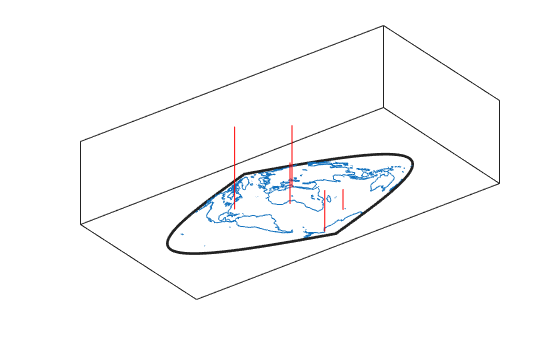# stem3m

Project stem plot on map axes

## Syntax

```h = stem3m(lat,lon,z) h = stem3m(lat,lon,z,LineType) h = stem3m(lat,lon,z,PropertyName,PropertyValue,...) ```

## Description

`h = stem3m(lat,lon,z)` displays a stem plot on the current map axes. Stems are located at the points (`lat`,`lon`) and extend from an altitude of 0 to the values of `z`. The coordinate inputs should be in the same `AngleUnits` as the map axes. It is important to note that the selection of z-values will greatly affect the 3-D look of the plot. Regardless of `AngleUnits`, the x and y limits of the map axes are at most -π to +π and -π/2 to +π/2, respectively. This means that for most purposes, appropriate `z` values would be on the order of 1 to 3, not 10 to 30. The axes `DataAspectRatio` property can be used to adjust the appearance of the graphic. The handles of the displayed stem lines can be returned in `h`.

`h = stem3m(lat,lon,z,LineType)` defines the style of the stem plot's lines, specified by a `linespec`.

`h = stem3m(lat,lon,z,PropertyName,PropertyValue,...)` allows any property/value pair recognized by the MATLAB® `line` function to be specified for the stems.

A stem plot displays data as lines extending normal to the xy-plane, in this case, on a map.

## Examples

collapse all

Project a stem plot on a map axes.

```load coastlines axesm sinusoid; view(3) h = framem; set(h,'zdata',zeros(size(coastlat))) plotm(coastlat,coastlon) ptlat = [0 30 30 -50 -78]'; ptlon = [0 30 -70 65 -35]'; ptz = [1 1.5 2 .5 1]'; stem3m(ptlat,ptlon,ptz,'r-')```